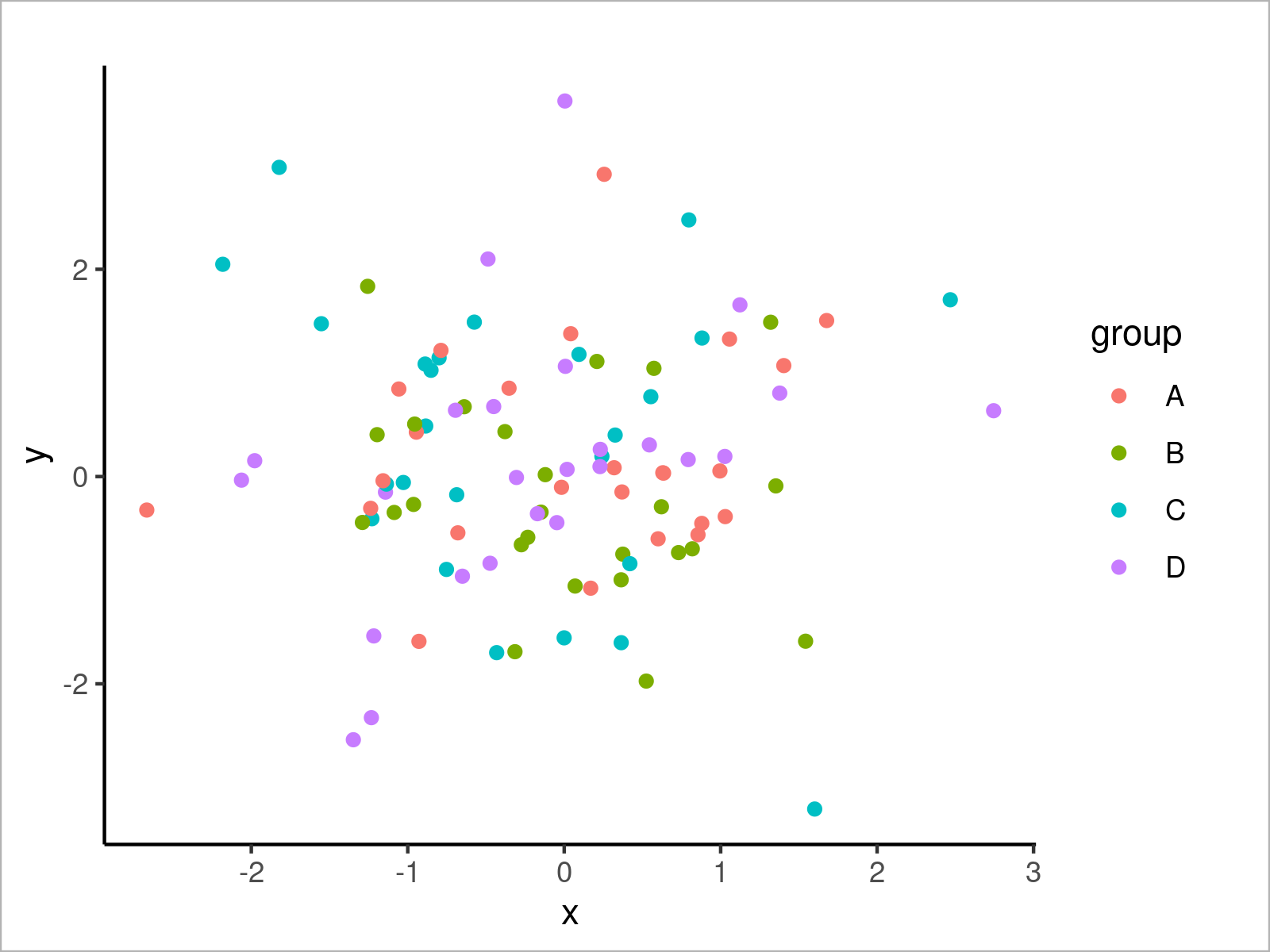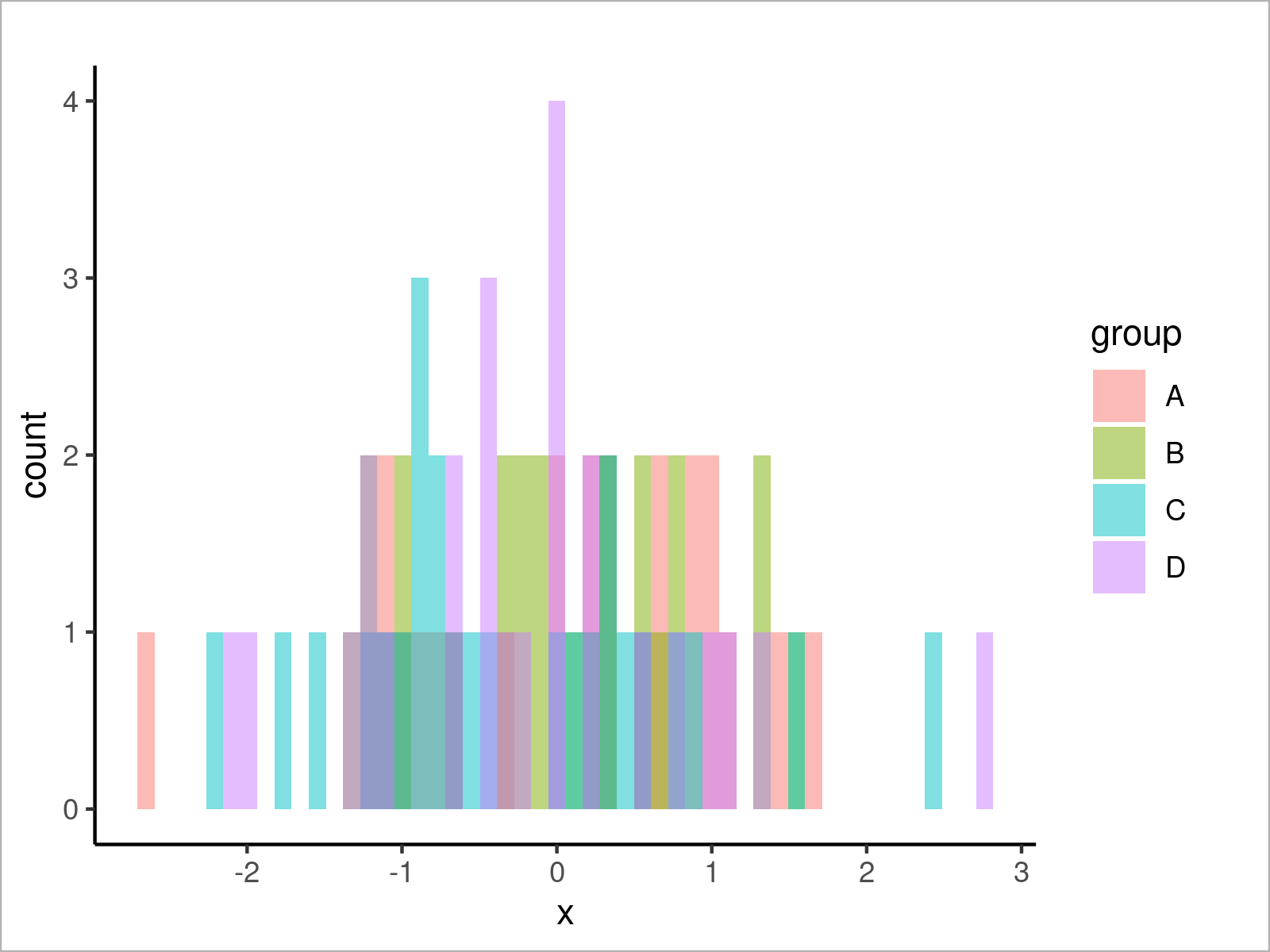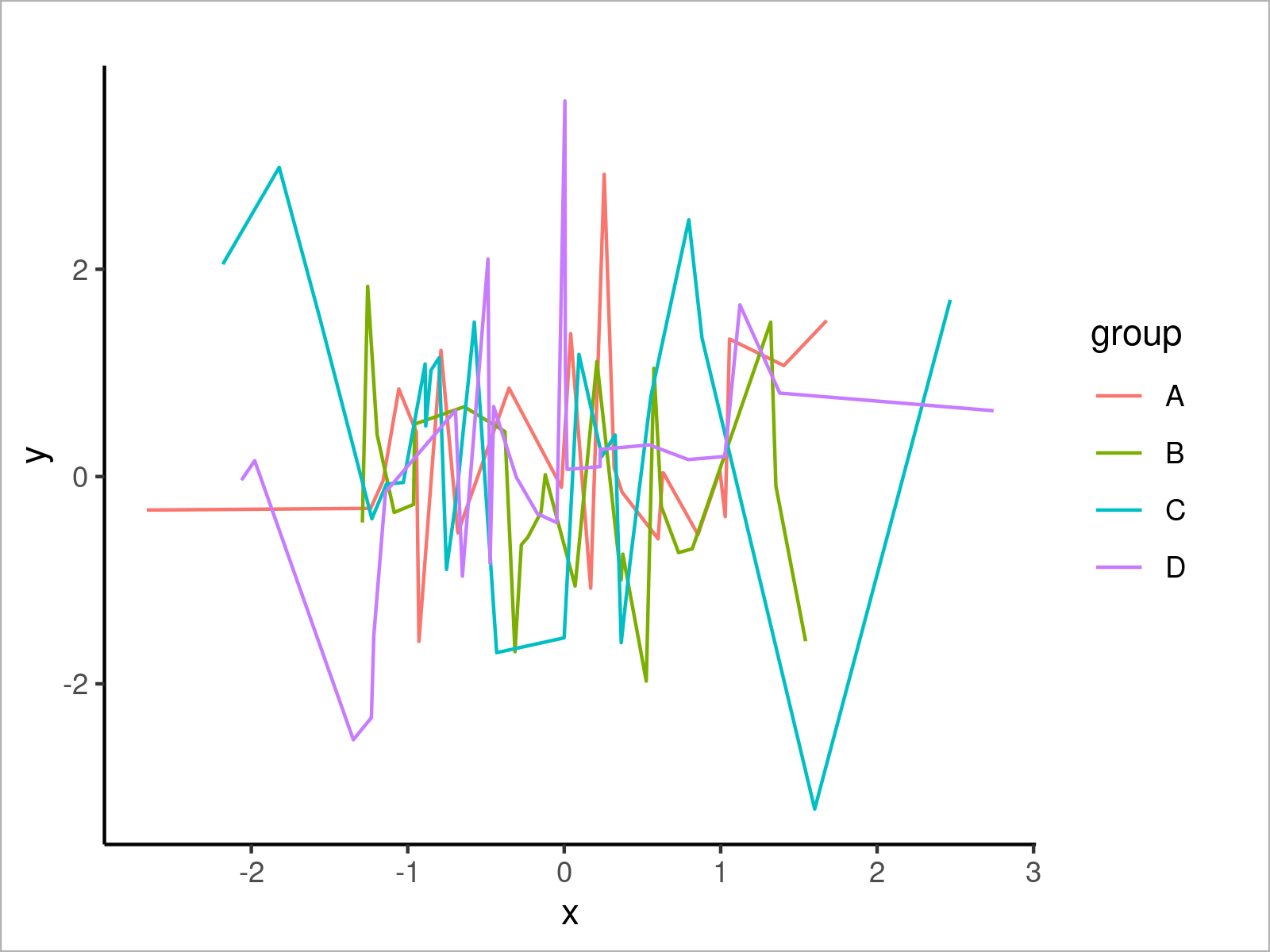# theme_classic ggplot2 Theme in R (6 Examples)

In this tutorial you’ll learn how to change the ggplot2 theme to the theme_classic in R programming.

The post is structured as follows:

It’s time to dive into the examples:

## Example Data & Software Packages

Let’s first create some example data:

```set.seed(84636)                            # Set random seed
data <- data.frame(x = rnorm(100),         # Create example data frame
y = rnorm(100),
group = LETTERS[1:4])Table 1 shows that our example data comprises three columns.

In order to use the functions of the ggplot2 software package, we also need to install and load ggplot2:

```install.packages("ggplot2")                # Install ggplot2 package

The following examples will use the classical style of the ggplot2 package to draw our data. Let’s do this!

## Example 1: Draw ggplot2 Scatterplot Using theme_classic()

```ggplot(data,                               # theme_classic scatterplot
aes(x = x,
y = y,
col = group)) +
geom_point() +
theme_classic()```## Example 2: Draw ggplot2 Density Plot Using theme_classic()

```ggplot(data,                               # theme_classic density plot
aes(x = x,
fill = group)) +
geom_density(alpha = 0.5) +
theme_classic()```## Example 3: Draw ggplot2 Histogram Using theme_classic()

```ggplot(data,                               # theme_classic histogram
aes(x = x,
fill = group)) +
geom_histogram(alpha = 0.5,
position = "identity",
bins = 50) +
theme_classic()```## Example 4: Draw ggplot2 Barchart Using theme_classic()

```ggplot(aggregate(y ~ group, data, sum),    # theme_classic barplot
aes(x = group,
y = y,
fill = group)) +
geom_bar(stat = "identity") +
theme_classic()```## Example 5: Draw ggplot2 Boxplot Using theme_classic()

```ggplot(data,                               # theme_classic boxplot
aes(x = x,
fill = group)) +
geom_boxplot() +
theme_classic()```## Example 6: Draw ggplot2 Line Plot Using theme_classic()

```ggplot(data,                               # theme_classic line plot
aes(x = x,
y = y,
col = group)) +
geom_line() +
theme_classic()```## Video & Further Resources

Have a look at the following video which I have published on my YouTube channel. In this tutorial, I give a detailed introduction to the ggplot2 Package and data visualization in R, structured in different sections with examples for beginners but also advanced users.

Please accept YouTube cookies to play this video. By accepting you will be accessing content from YouTube, a service provided by an external third party.If you accept this notice, your choice will be saved and the page will refresh.

On Statistics Globe, you can also find articles on how to construct graphics using different ggplot2 themes:

Furthermore, you may have a look at the introductions to other packages that provide additional themes for ggplot2 plots:

In addition, you might have a look at the related articles on my homepage:

In this post, I have demonstrated how to transform the ggplot2 theme to the theme_classic in the R programming language. In case you have further questions or comments, let me know in the comments section.

Subscribe to the Statistics Globe Newsletter